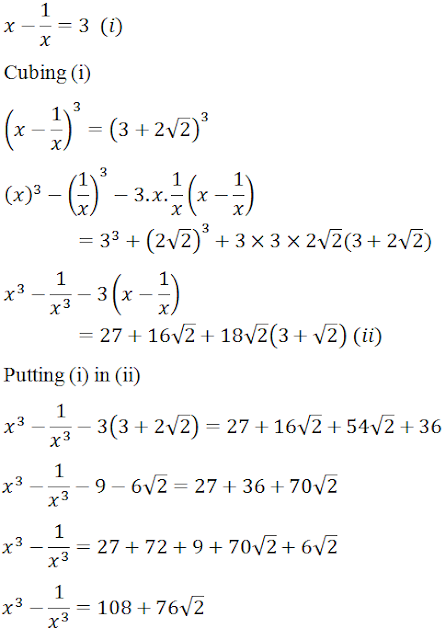#### Chapter 4 Algebraic Identities R.D. Sharma Solutions for Class 9th Math Exercise 4.3

Exercise 4.3

1. Find the cube of each of the following binomials expressions: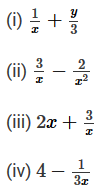Solution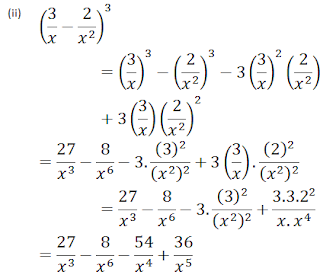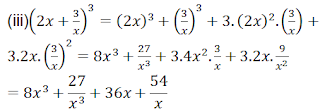2. Simplify each of the following: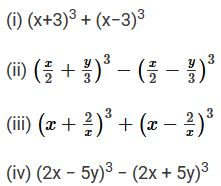Solution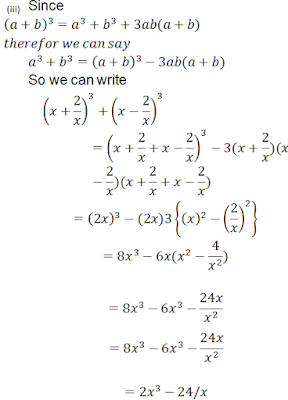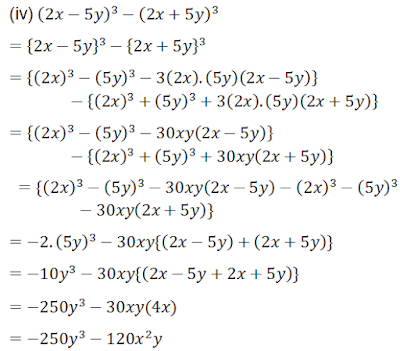3. If a + b = 10 and ab = 21, find the value of a3 + b3

Solution4.  If a − b = 4 and ab = 21, find the value of a3 −b3

Solution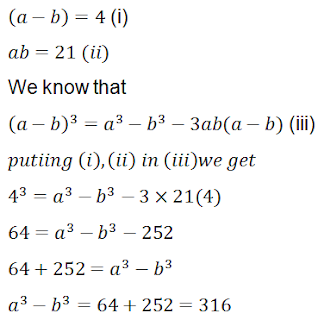5. If x+1x=5$x+\frac{1}{x}=5$, find the value of x3+1x3

Solution6. If x1x=7 find the value of x31x3

Solution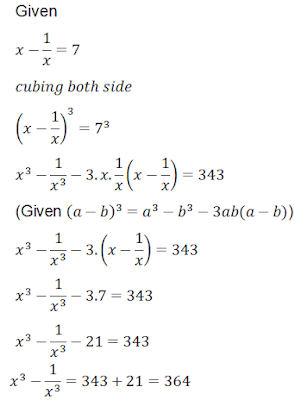Solution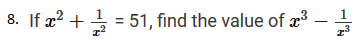Solution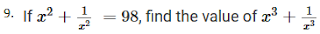Solution10.  If 2x+3y = 13 and xy = 6, find the value of 8x3 + 27y3

Solution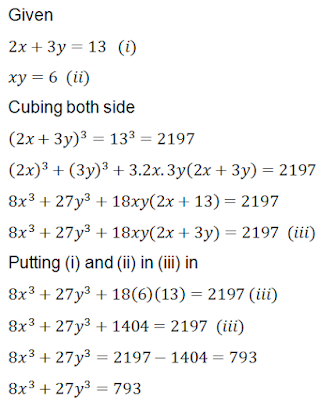11. If 3x − 2y = 11 and xy = 12, find the value of 27x3 − 8y3

Solution12. If x4+1x4= 119, find the value of x31x3

Solution13.  Evaluate each of the following:
(i) (103)3
(ii) (98)3
(iii) (9.9)3
(iv) (10.4)3
(v) (598)3
(vi) (99)3

Solution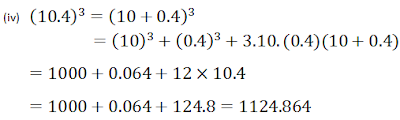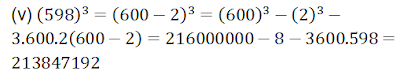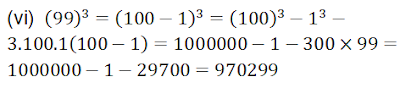14 . Evaluate each of the following:
(i) 1113 − 893
(ii) 463+343
(iii) 1043 + 963
(iv) 933 − 1073

Solution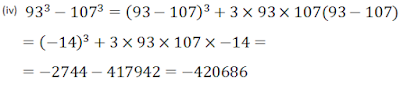SolutionSolution17. Find the value of 27x3 + 8y3, if
(i) 3x + 2y = 14 and xy = 8
(ii) 3x + 2y = 20 and xy = 149

Solution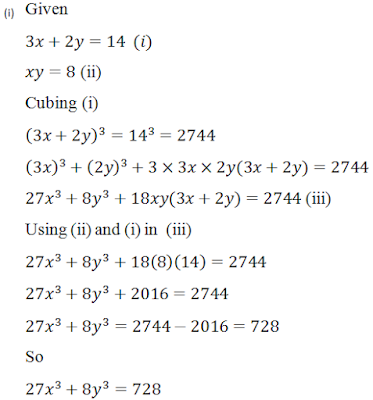(ii)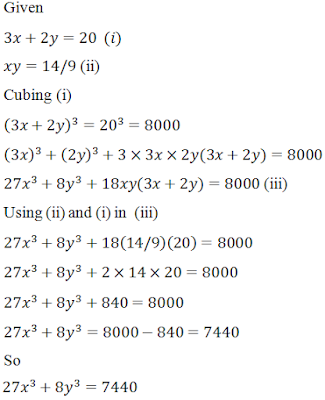18. Find the value of 64x3 − 125z3, if 4x − 5z = 16 and xz = 12.

Solution19. If x1x=3+22$x-\frac{1}{x}=3+2\sqrt{2}$, find the value of x31x3

Solution